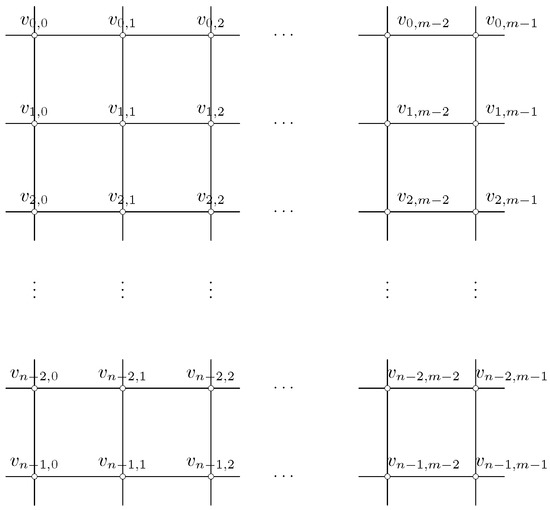Next Article in Journal
A New Generalized Taylor-Like Explicit Method for Stiff Ordinary Differential Equations
Previous Article in Journal
Inframarginal Model Analysis of the Evolution of Agricultural Division of Labor
Open AccessArticle

# The k-Rainbow Domination Number of Cn□Cm

byHong Gao 1,*,Kun Li 1 andYuansheng Yang 2
1
College of Science, Dalian Maritime University, Dalian 116026, China
2
School of Computer Science and Technology, Dalian University of Technology, Dalian 116024, China
*
Author to whom correspondence should be addressed.
Mathematics 2019, 7(12), 1153; https://doi.org/10.3390/math7121153
Received: 10 October 2019 / Revised: 20 November 2019 / Accepted: 21 November 2019 / Published: 1 December 2019
Given a graph G and a set of k colors, assign an arbitrary subset of these colors to each vertex of G. If each vertex to which the empty set is assigned has all k colors in its neighborhood, then the assignment is called a k-rainbow dominating function (kRDF) of G. The minimum sum of numbers of assigned colors over all vertices of G is called the k-rainbow domination number of graph G, denoted by $γ r k ( G )$ . In this paper, we focus on the study of the k-rainbow domination number of the Cartesian product of cycles, $C n □ C m$ . For $k ≥ 8$ , based on the results of J. Amjadi et al. (2017), $γ r k ( C n □ C m ) = m n$ . For $( 4 ≤ k ≤ 7 )$ , we give a proof for the new lower bound of $γ r 4 ( C n □ C 3 )$ . We construct some novel and recursive kRDFs which are good enough and upon these functions we get sharp upper bounds of $γ r k ( C n □ C m )$ . Therefore, we obtain the following results: (1) $γ r 4 ( C n □ C 3 ) = 2 n$ ; (2) $γ r k ( C n □ C m ) = k m n 8$ for $n ≡ 0 ( mod 4 ) , m ≡ 0 ( mod 4 )$ $( 4 ≤ k ≤ 7 )$ ; (3) for $n ≢ 0 ( mod 4 )$ or $m ≢ 0 ( mod 4 )$ , $m n 2 ≤ γ r 4 ( C n □ C m ) ≤ m n 2 + m + n 2 − 1$ and $k m n 8 ≤ γ r k ( C n □ C m ) ≤ k m n + n 8 + m$ for $5 ≤ k ≤ 7$ . We also discuss Vizing’s conjecture on the k-rainbow domination number of $C n □ C m$ . View Full-Text
Show FiguresFigure 1

MDPI and ACS Style

Gao, H.; Li, K.; Yang, Y. The k-Rainbow Domination Number of CnCm. Mathematics 2019, 7, 1153.